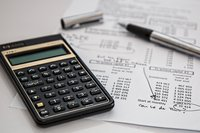# Maths

## Maths is the Language of the universe and a hallmark of successMathematics is essential for everyday life and understanding our world. At Thomas Becket Catholic School, we strive to develop accurate, efficient, and flexible problem solvers to increase their reasoning abilities. This will be done by providing authentic and rigorous problem-solving opportunities that lead to engaging student-to-student discussions facilitated by teachers through carefully planned questions.

The Mathematics department believes in the potential of every student; hence the vision of the department is to develop the mindset and mathematical skills which will enable students to select the correct process to assess and solve mathematical problems.

By building a sense of trust, love and respect within the department, students will achieve qualifications to pursue a fulfilling career in order to contribute positively to their communities and be active, global citizens.

Students will develop the skills to use digital tools appropriately to explore mathematical problems.

In the Mathematics department, every student matters and we continue to strive to help students achieve their very best through excellent learning and teaching which links to our mission and vision of the school.

## Year 7

• Number skills, Decimals and measures, Expressions functions and formulae, Analysing and displaying data
• Fractions and percentages, Probability, Ratio and proportion
• Lines and angles, Sequencing and graphs, Transformations

## Year 8

• Number, Area and volume, Statistics, graphs and charts, Expressions and equations
• Real-life graphs, Decimals and ratio, Lines and angles
• Calculating with fractions, Percentages, decimals and fractions, Straight-line graph

## Year 9

• Numbers
• Algebra
• Interpreting and representing data
• Fractions, ratios and percentages
• Angles and trigonometry
• Graphs
• Multiplicative reasoning

## Year 10

• Transformations and constructions
• Equations and inequalities
• Equations and graphs
• Similarity and congruence
• Perimeter, Area and Volume
• Statistics/Average and Range
• Probability
• Circle theorem     (H)
• Ratio and Proportion (F)
• More trigonometry (H)